## Approximate Functions with a Direct Lookup Table

Using the Lookup Table Optimizer, you can generate a direct lookup table approximating a Simulink® block, or a function. Direct lookup tables are efficient to implement on hardware because they do not require any calculations.

### Generate a Two-Dimensional Direct Lookup Table Approximation

Create a `FunctionApproximation.Problem` object specifying the function for which to generate the approximate. To generate a direct lookup table, set the interpolation method to `None` in the `FunctionApproximation.Options` object.

```problem = FunctionApproximation.Problem('atan2'); problem.InputTypes = [numerictype(0,4,2) numerictype(0,8,4)]; problem.OutputType = fixdt(0,8,7); problem.Options.Interpolation = "None"; problem.Options.AbsTol = 2^-4; problem.Options.RelTol = 0; problem.Options.WordLengths = 1:8; ```

Use the `solve` method to generate the optimal lookup table.

```solution = solve(problem) ```
```| ID | Memory (bits) | Feasible | Table Size | Intermediate WLs | TableData WL | Error(Max,Current) | | 0 | 32768 | 1 | [16 256] | [4 8] | 8 | 6.250000e-02, 3.902460e-03 | | 1 | 28672 | 1 | [16 256] | [4 8] | 7 | 6.250000e-02, 7.811287e-03 | | 2 | 24576 | 1 | [16 256] | [4 8] | 6 | 6.250000e-02, 1.561990e-02 | | 3 | 16384 | 1 | [16 128] | [4 7] | 8 | 6.250000e-02, 6.242016e-02 | | 4 | 14336 | 1 | [16 128] | [4 7] | 7 | 6.250000e-02, 5.707978e-02 | | 5 | 12288 | 1 | [16 128] | [4 7] | 6 | 6.250000e-02, 5.870371e-02 | | 6 | 10240 | 0 | [16 128] | [4 7] | 5 | 6.250000e-02, 8.585766e-02 | | 7 | 8192 | 0 | [16 128] | [4 7] | 4 | 6.250000e-02, 1.020576e-01 | Best Solution | ID | Memory (bits) | Feasible | Table Size | Intermediate WLs | TableData WL | Error(Max,Current) | | 5 | 12288 | 1 | [16 128] | [4 7] | 6 | 6.250000e-02, 5.870371e-02 | solution = 1x1 FunctionApproximation.LUTSolution with properties: ID: 5 Feasible: "true" ```

Use the `compare` method to compare the output of the original function and the approximate.

```compare(solution); ```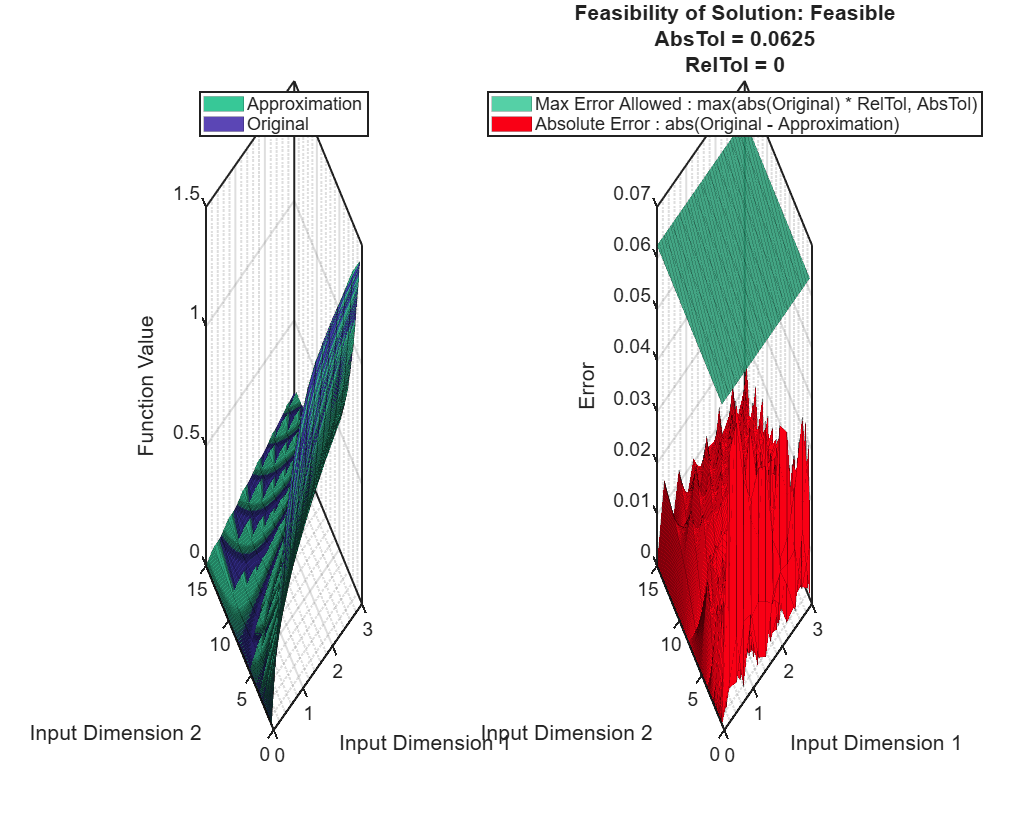Use the `approximate` method to generate a Simulink™ subsystem containing the generated direct lookup table.

```approximate(solution) ```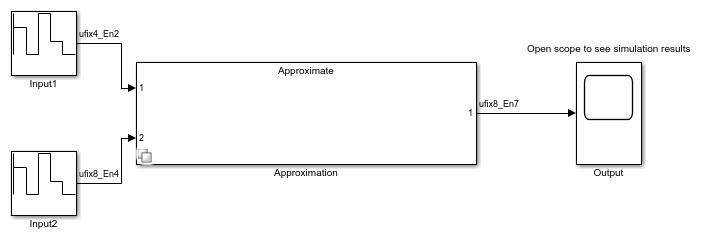### Generate a Direct Lookup Table Approximation for a Subsystem

This example shows how to approximate a Simulink™ subsystem with a direct lookup table.

Open the model containing the subsystem to approximate.

```functionToApproximate = 'ex_direct_approximation/MathExpression'; open_system('ex_direct_approximation'); ```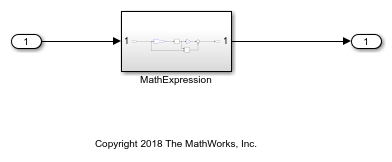To generate a direct lookup table, set the interpolation method to `None`.

```problem = FunctionApproximation.Problem(functionToApproximate); problem.Options.Interpolation = 'None'; problem.Options.RelTol = 0; problem.Options.AbsTol = 0.2; problem.Options.WordLengths = [7 8 9 16]; solution = solve(problem); ```
```| ID | Memory (bits) | Feasible | Table Size | Intermediate WLs | TableData WL | Error(Max,Current) | | 0 | 2097152 | 1 | 65536 | 16 | 32 | 2.000000e-01, 0.000000e+00 | | 1 | 896 | 0 | 128 | 7 | 7 | 2.000000e-01, 2.265625e+00 | | 2 | 1024 | 0 | 128 | 7 | 8 | 2.000000e-01, 2.265625e+00 | | 3 | 1152 | 0 | 128 | 7 | 9 | 2.000000e-01, 2.265625e+00 | | 4 | 2048 | 0 | 128 | 7 | 16 | 2.000000e-01, 2.267090e+00 | Best Solution | ID | Memory (bits) | Feasible | Table Size | Intermediate WLs | TableData WL | Error(Max,Current) | | 0 | 2097152 | 1 | 65536 | 16 | 32 | 2.000000e-01, 0.000000e+00 | ```

Compare the original subsystem behavior to the lookup table approximation.

```compare(solution); ```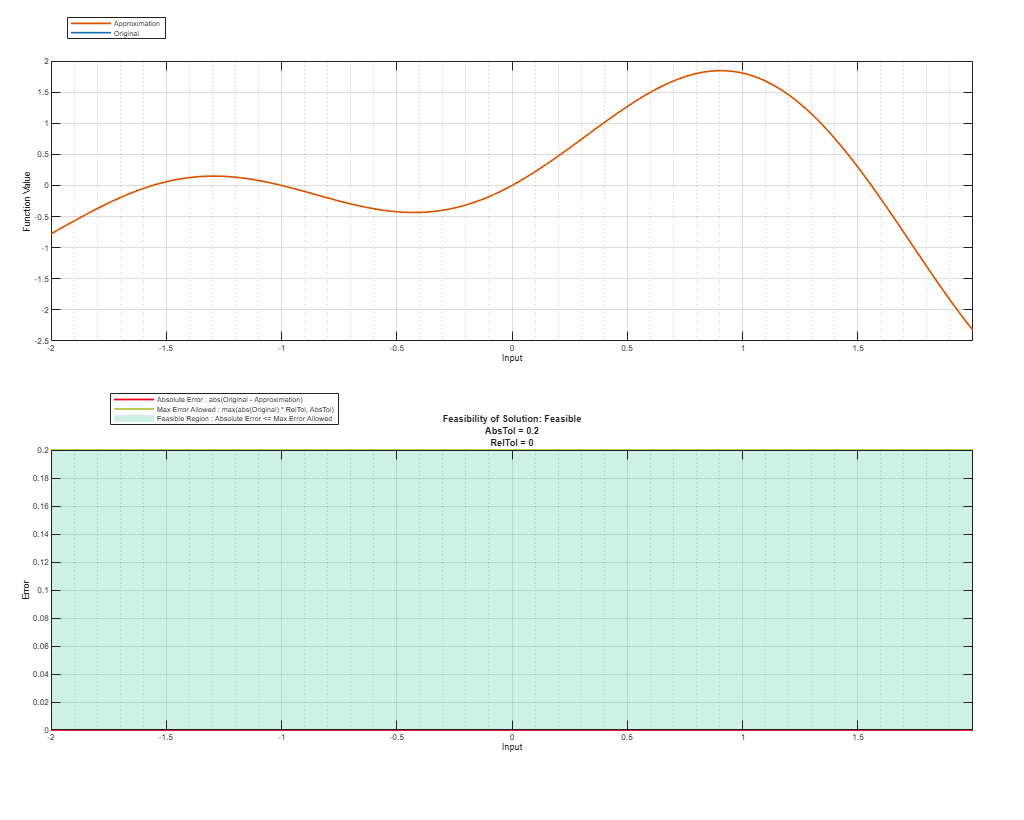Generate a new subsystem containing the lookup table approximation.

```approximate(solution); ```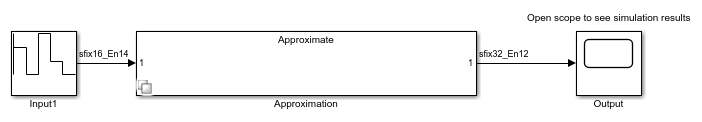Replace the original subsystem with the new subsystem containing the lookup table approximation.

```replaceWithApproximate(solution); ```

You can revert your model back to its original state using the `revertToOriginal` function. This function places the original subsystem back into the model.

```revertToOriginal(solution); ```

## Support